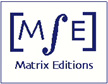Matrix Editions
serious mathematics,
written with the reader in mind

# Future Books

## Volume 3: Manifolds that Fiber over the Circle

##### by John H. Hubbard

Would you like to be notified when this book is published?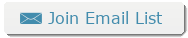We hope that this book will be available by June 2019. Here we give the opening paragraphs of each chapter, as they now stand, and some figures. In the book, labels are included in the figures, using "puts". Placement of the figures here does not reflect their placement in the book.

#### Chapter 11 Geometry of hyperbolic space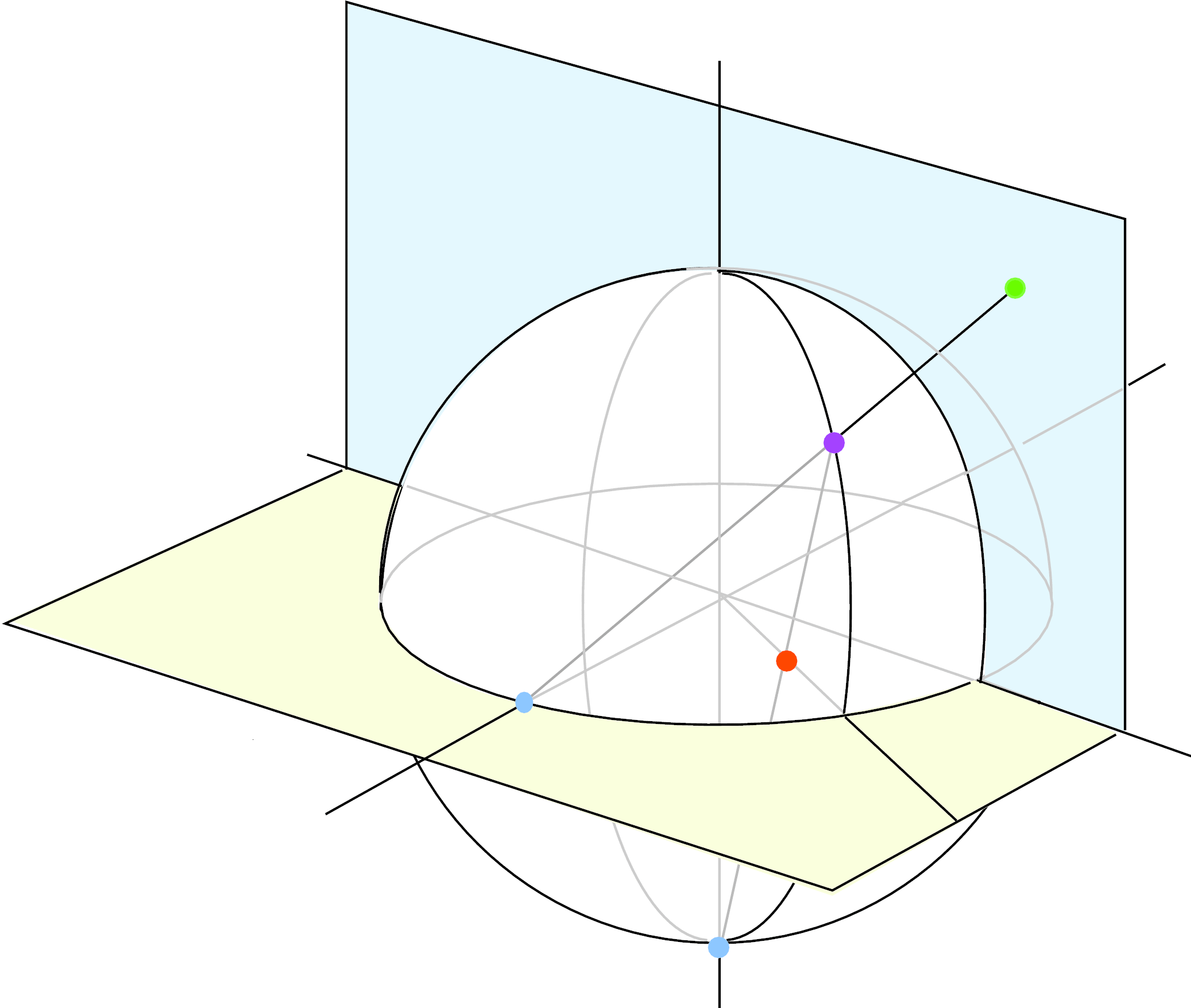In this chapter we give a short introduction to the geometry of hyperbolic space and to Kleinian groups. We begin in Sections 11.1-11.3 with hyperbolic space and its group of automorphisms. In Section 11.4 we discuss elementary and non-elementary Kleinian groups. Section 11.5 defines the limit set of a Kleinian group and gives some of its basic properties.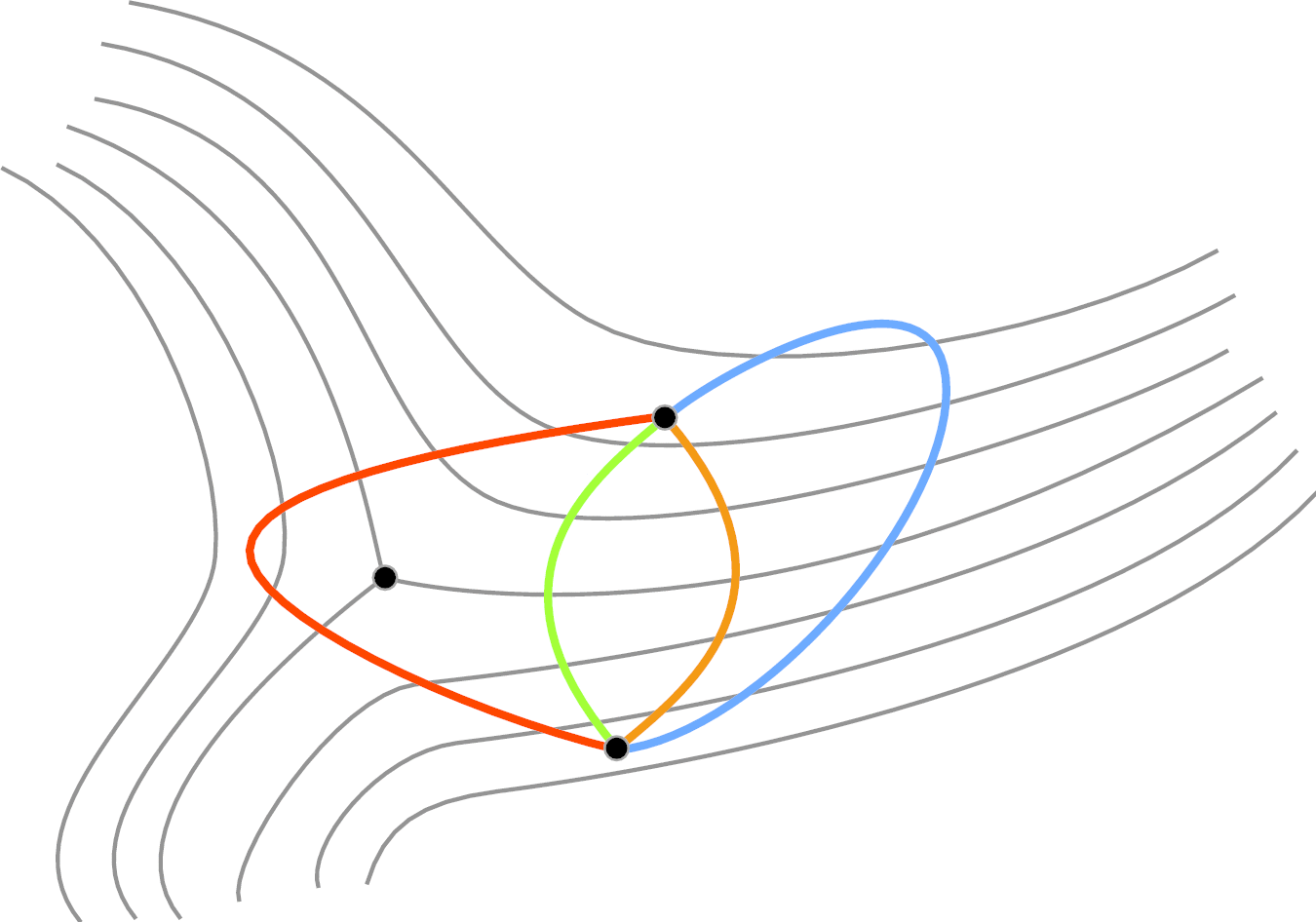We go on in Section 11.6 to the Jørgensen inequality, a beautiful and rather mysterious inequality that exploits the discreteness of a Kleinian group in an essential way. This makes it possible to give in Section 11.7 a fairly easy proof of the Margulis lemma. This is a 3-dimensional analogue of the collaring theorem (Theorem 3.8.3 in Volume 1), giving a complete description of the thin parts of a hyperbolic 3-manifold; it provides an analogue of the plumbing picture of hyperbolic surfaces given in Section 3.8.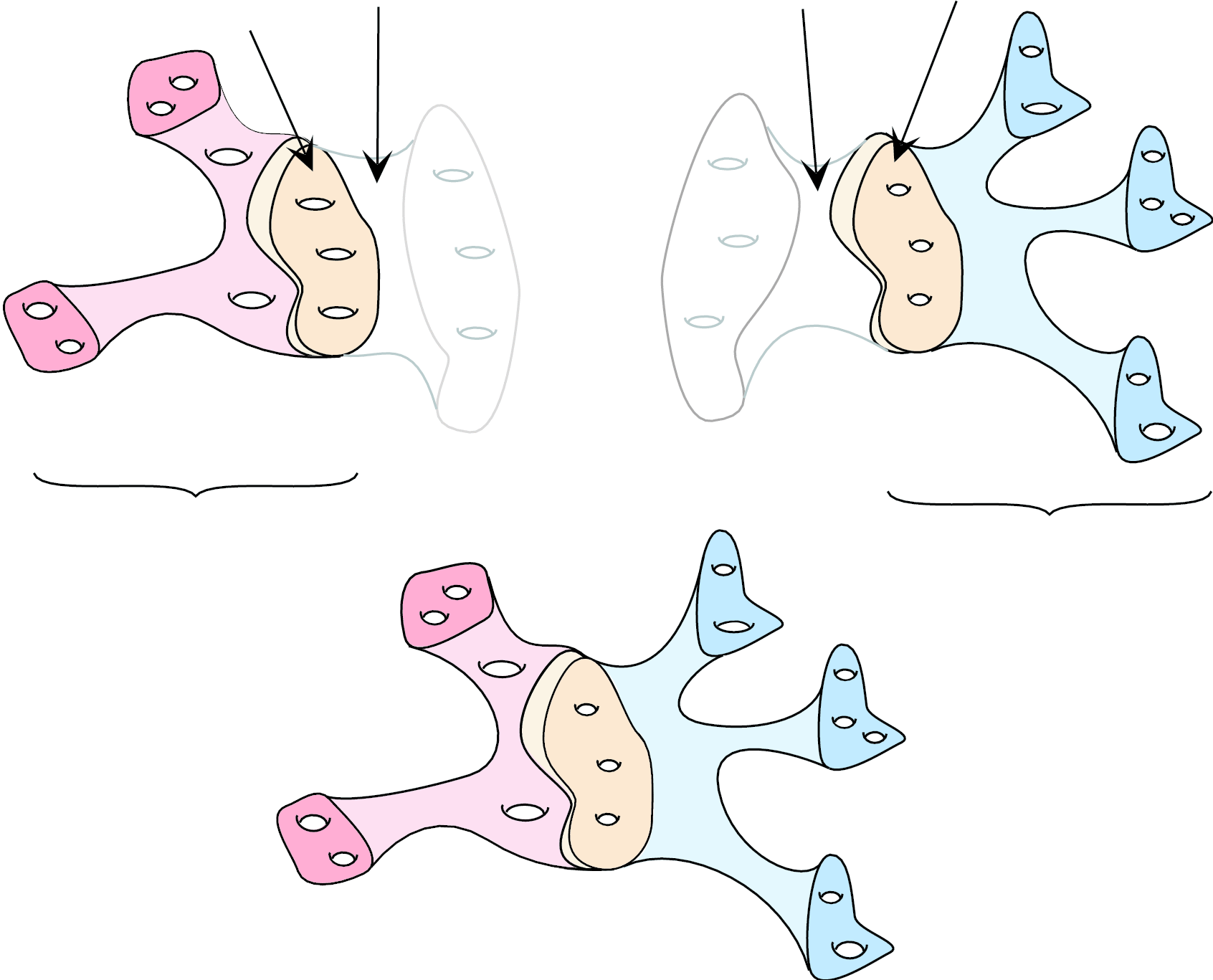We find the proof of Jørgensen's inequality hard to motivate, so we also give in Appendix D2 a different proof of the Margulis lemma, which is cruder, but much easier to understand.

Thurston's hyperbolization theorems require thinking not just about individual Kleinian groups, but also about their limits. There are two notions of "limit of a sequence of Kleinian groups'', with surprisingly different properties: algebraic limits, which are relatively easy to understand, and geometric limits, which can be amazingly complicated. Algebraic limits are described in Section 11.8, geometric limits in Section 11.9.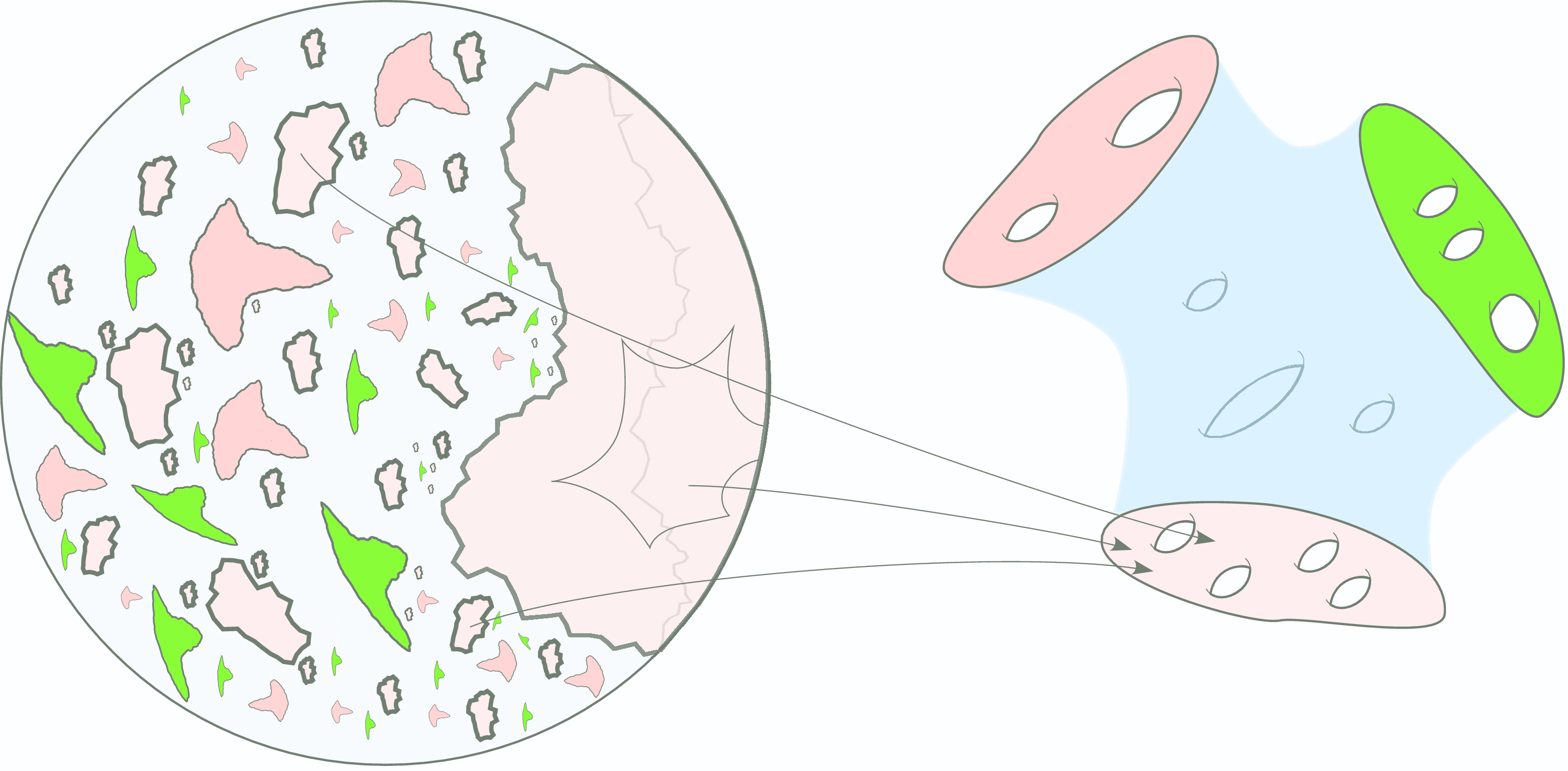Thurston's second hyperbolization theorem requires the Klein-Maskit combination theorems, which will eventually allow us to glue together hyperbolic manifolds. These theorems,  proved in Section 11.10 also illustrate the power of 3-dimensional thinking about Kleinian groups; it is quite hard to see why the combined groups as defined are discrete if one sticks to the action on the Riemann sphere.
Finally, in Section 11.11,we prove the 3-dimensional analogue of the Poincaré polygon theorem.

#### Chapter 12 Rigidity theorems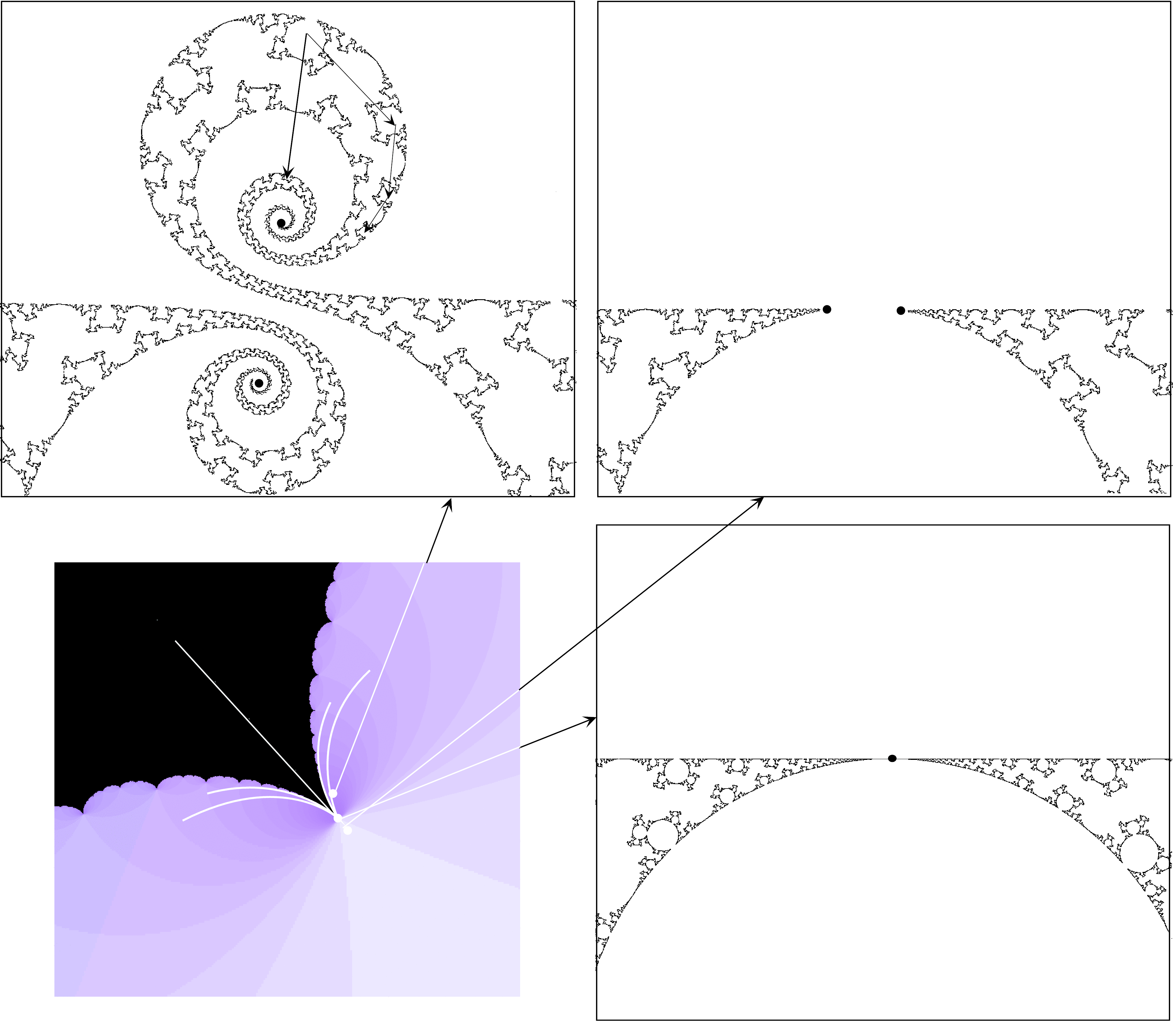In the first half of this chapter we prove three great theorems, due to Ahlfors, McMullen, and Mostow. Although the statements look quite different, they are tech­nically closely related: they are all concerned with the construction of Beltrami forms on partial overline H3 invariant under a Kleinian group. Since these theorems show that such Beltrami forms are very restricted, they are called rigidity theorems

In the second half (Sections 12.5-12.8) we prove that the central hypothesis of McMullen's rigidity theorem holds for quasi-Fuchsian groups. We need this to prove the hyperbolization theorem for 3-manifolds that fiber over the circle. Along the way we develop several topics, including laminations and pleated surfaces,of great interest in their own right.

#### Chapter 13 Hyperbolization of 3-manifolds that fiber over the circle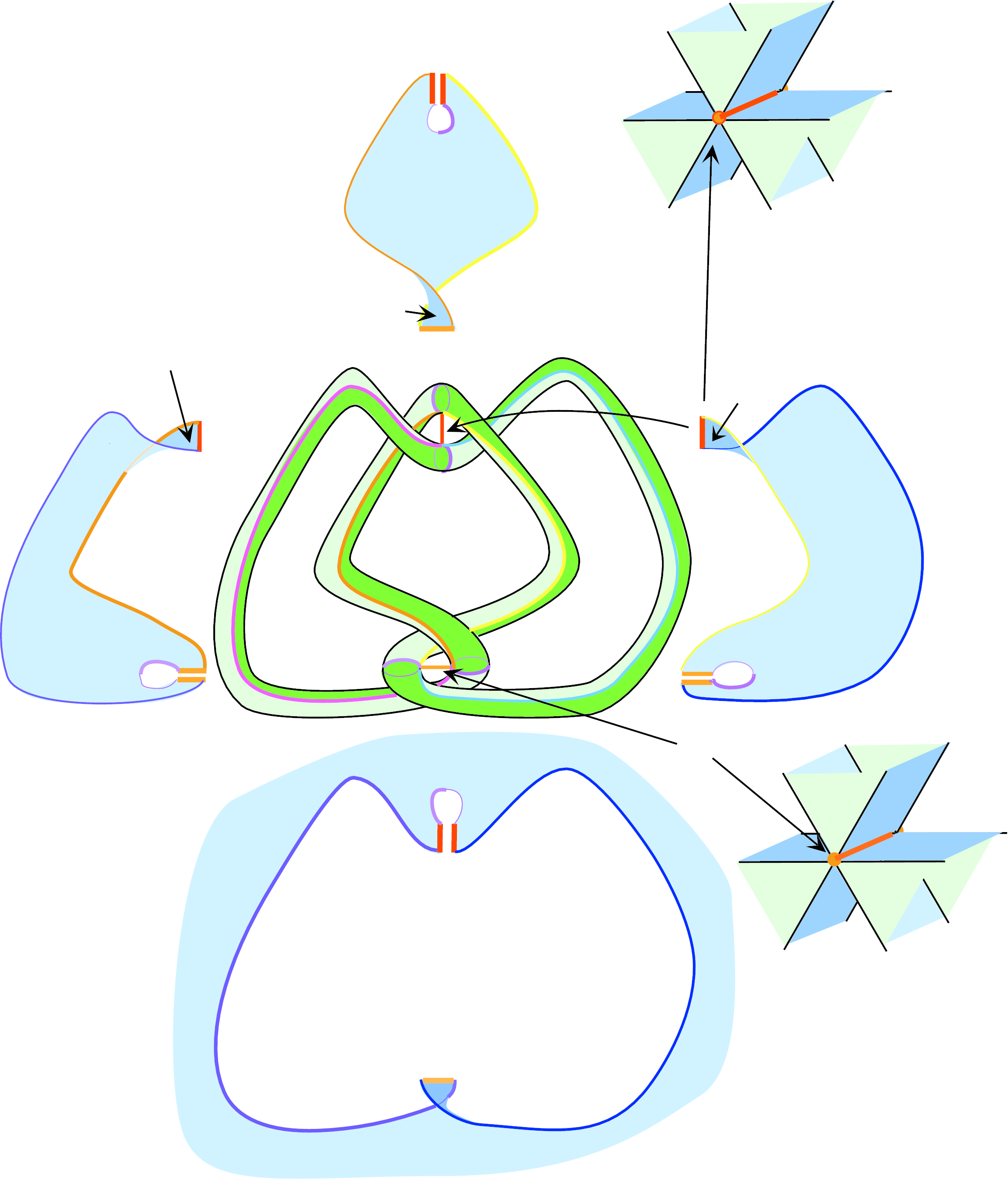One of the great accomplishments of nine­teenth century math­ema­tics was showing that all surfaces of genus g >= 2 admit hyperbolic structures. We now present a 3-dimensional version of this theorem: Theorem 13.0.1. This is one of the great accomplishments of twentieth century math­ema­tics.

Let S =\overline S-Z be an orientable surface with an orientable surface with overline S compact and Z finite. Let f : S to S be an orientation-preserving  homeomorphism. Denote by Mf the mapping torus of f , i.e., the quotient of [0,1] x S by the equivalence relation that identifies (0, x) to (1, f(x)). Every 3-manifold that fibers over the circle is of this form; the corresponding map f is called the holonomy map. It is defined only up to isotopy and so is really an element of the mapping class group MCG(S).

Theorem 13.0.1 (Hyperbolization of 3-manifolds that fiber over the circle) The 3-manifold Mf admits a complete hyperbolic structure if and only if the holonomy map f is homotopic to a pseudo-Anosov homeomorphism.

Proving this theorem will take the entire chapter.

#### Appendices

Appendix D1  The Nullstellensatz
Appendix D3  The Margulis lemma: another proof
Appendix D3  Amalgamated sums
Appendix D4  Arithmetic Kleinian groups
Appendix D5  Ergodic flow and Mostow rigidity
Appendix D6  Sullivan's rigidity theorem
Appendix D7  Positivity of the Riemann matrix